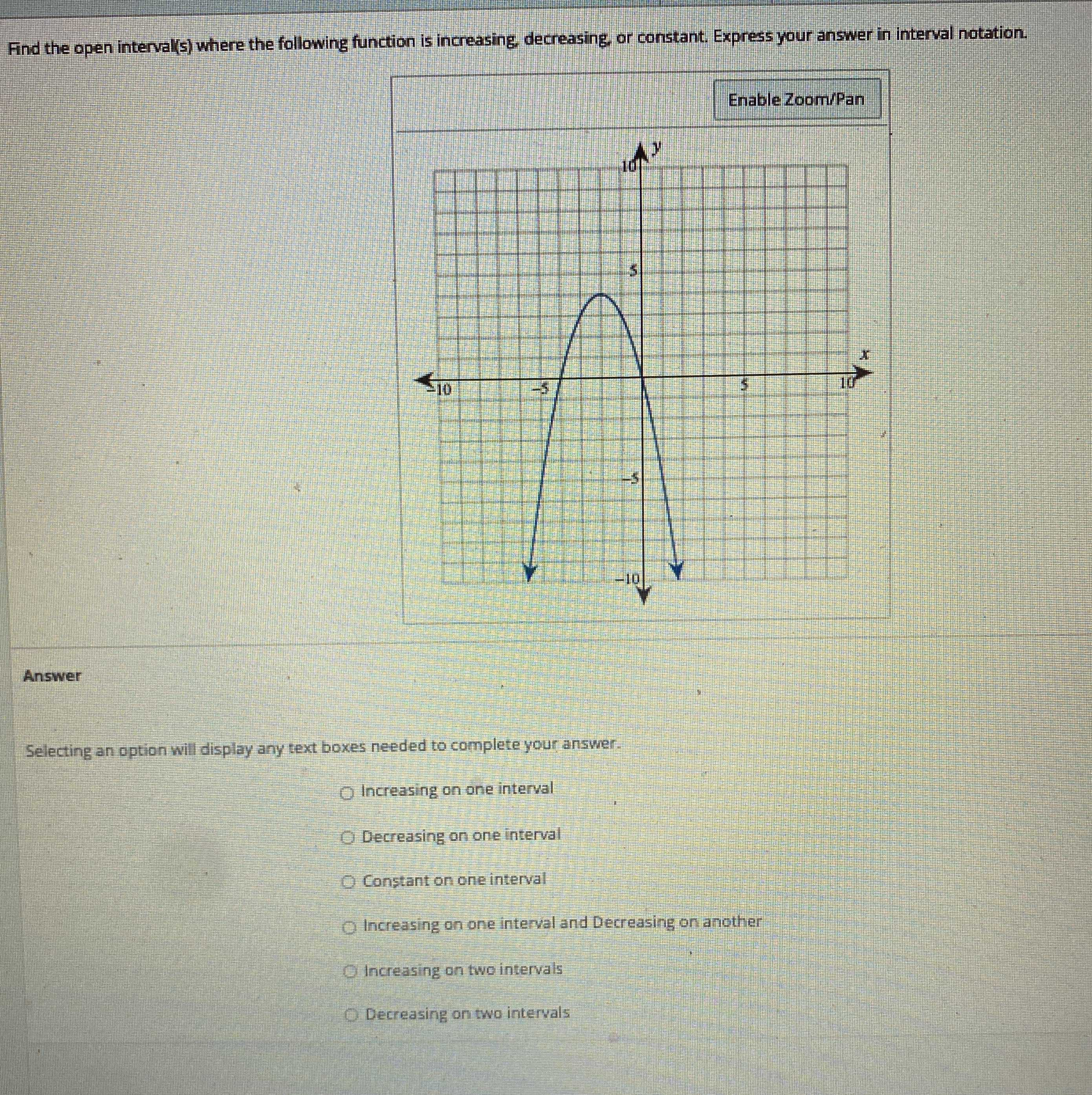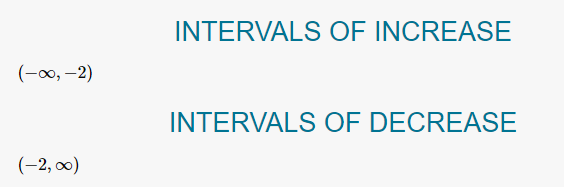### Still have math questions?

Algebra
QuestionFind the open interval(s) where the following function is increasing, decreasing, or constant. Express your answer in interval notation.

Selecting an option wil display any text boxes needed to complete your answer.

Increasing on one interval

Decreasing on one interval

Constant on one interval

Increasing on one interval and Decreasing on another

Increasing on two intervals

Decreasing on two intervals